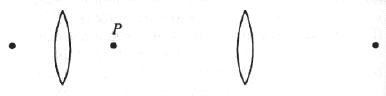GR 8677927796770177 | # Login | Register

GR9277 #22
Problem
 GREPhysics.NET Official Solution Alternate Solutions
\prob{22}A simple telescope consists of two convex lenses, the objective and the eypiece, which have a common focal point P as shown in the figure above. If the focal length of the objective is 1.0 m and the angular magnification of the telescope is 10, what is the optical path length between objective and eyepieece?

1. 0.1m
2. 0.9m
3. 1.0m
4. 1.1m
5. 10m

Optics}Telescope

The magnification for a telescope is related to the focal length for the eyepiece and objective by . (Note that it is the eye-piece that magnifies it. The objective merely sends an image that's within view of the eye-piece. However, magnification is inversely related to focal length.)

The problem gives angular magnification to be . The distance between the objective and eyepiece is the sum of the focal lengths (since the light comes from infinity). m as in choice (D).Alternate Solutions
 There are no Alternate Solutions for this problem. Be the first to post one!
kstephe6
2012-11-05 09:33:02
The faster the answer the better.

the distance is the sum of the two focal lengths, so it must be between 1 and 2 meters. Choice D. DONE
 djh1012014-08-29 16:06:32 You win.
 hipparcos2018-04-05 01:57:20 Nice one!sky
2009-11-06 13:14:32
This can also be solved by similar triangles. Draw 2 rays passing through the common focal point of the two lenses and ending in the center of each lens. This will give two similar triangles that share equal vertical angles at the focus. the magnification factor tells us that the base of the triangle touching the objective lens should have a base length of 10 units. They give its height as the longer focal length of 1.0m. The smaller eyepiece should have a base length of 1 unit, again due to the magnification. let x be the focal length of the eyepiece. The similar triangle proportion yields,

=

or,

x=0.1m

addition of the focal lengths gives 1.1m (D)

now that I wrote it out I think this is probably the derivation of the equation used in the solution already given. lolemailzac
2007-11-02 18:39:17
Since the distance between the two eye pieces is the sum of the focal lengths, you know it can't be A B or C, and and the focal length of the eyepiece is usually smaller than that of the objective (at least a can't think of a case when its not) so you know the answer has to be less than 2, hence D.LaTeX syntax supported through dollar sign wrappers $, ex.,$\alpha^2_0$produces . type this... to get...$\int_0^\infty\partial\Rightarrow\ddot{x},\dot{x}\sqrt{z}\langle my \rangle\left( abacadabra \right)_{me}\vec{E}\frac{a}{b}\$